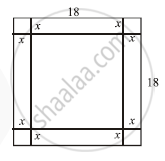# An Open Box is to Be Made Out of a Piece of a Square Card Board of Sides 18 cms. by Cutting off Equal Squares From The Comers and Tumi 11g up the Sides. Find the Maximum Volume of the Box. - Mathematics and Statistics

Sum

An open box is to be made out of a piece of a square card board of sides 18 cms. by cutting off equal squares from  the comers and turning up the sides. Find the maximum volume of the box.

#### SolutionLet each side of the square cut off from each corner be x cm.
Then the base of the box will be of side 18 - 2x cm and the height of the box will be x cm
Then volume of box V = ( 18 - 2x )( 18 - 2x )
V = ( 18 - 2x )2x
V = 4x3 + 324x - 72x2                             ..(i)
Differentiating w.r t to x , we get
(dV)/dx = 12x^2 + 324 - 144x

(dV)/dx = 12(x^2 - 12x + 27)               ...(ii)
For maximum volume (dV)/dx = 0
⇒ 12( x2 - 12x + 27 ) = 0
⇒ x2 - 9x -3x + 27 = 0
⇒ ( x - 9 )( x - 3 ) = 0
⇒ x = 9, 3
Again differentiating, we get
(d^2V)/dx^2 = 2x - 12                         ....(iii)
At x = 9.
(d^2V)/dx^2 = +ve
V is minimum at x = 9 at x = 3.
(d^2V)/dx^2 = - ve
V is maximum at x = 3.
Maximum volume V =
= 12 x 12 x 3 = 432 cm3

Concept: Maxima and Minima
Is there an error in this question or solution?